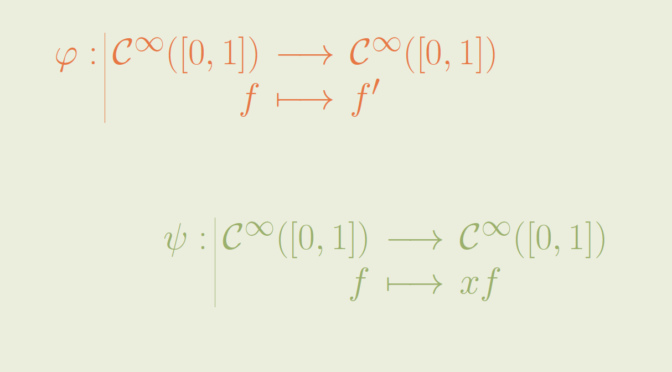# A linear map having all numbers as eigenvalue

Consider a linear map $$\varphi : E \to E$$ where $$E$$ is a linear space over the field $$\mathbb C$$ of the complex numbers. When $$E$$ is a finite dimensional vector space of dimension $$n \ge 1$$, the number of eigenvalues is finite. The eigenvalues are the roots of the characteristic polynomial $$\chi_\varphi$$ of $$\varphi$$. $$\chi_\varphi$$ is a complex polynomial of degree $$n \ge 1$$. Therefore the set of eigenvalues of $$\varphi$$ is non-empty and its cardinal is less than $$n$$.

Things are different when $$E$$ is an infinite dimensional space.

### A linear map having all numbers as eigenvalue

Let’s consider the linear space $$E=\mathcal C^\infty([0,1])$$ of smooth complex functions having derivatives of all orders and defined on the segment $$[0,1]$$. $$E$$ is an infinite dimensional space: it contains all the polynomial maps.

On $$E$$, we define the linear map $\begin{array}{l|rcl} \varphi : & \mathcal C^\infty([0,1]) & \longrightarrow & \mathcal C^\infty([0,1]) \\ & f & \longmapsto & f^\prime \end{array}$

The set of eigenvalues of $$\varphi$$ is all $$\mathbb C$$. Indeed, for $$\lambda \in \mathbb C$$ the map $$t \mapsto e^{\lambda t}$$ is an eigenvector associated to the eigenvalue $$\lambda$$.

### A linear map having no eigenvalue

On the same linear space $$E=\mathcal C^\infty([0,1])$$, we now consider the linear map $\begin{array}{l|rcl} \psi : & \mathcal C^\infty([0,1]) & \longrightarrow & \mathcal C^\infty([0,1]) \\ & f & \longmapsto & x f \end{array}$

Suppose that $$\lambda \in \mathbb C$$ is an eigenvalue of $$\psi$$ and $$h \in E$$ an eigenvector associated to $$\lambda$$. By hypothesis, there exists $$x_0 \in [0,1]$$ such that $$h(x_0) \neq 0$$. Even better, as $$h$$ is continuous, $$h$$ is non-vanishing on $$J \cap [0,1]$$ where $$J$$ is an open interval containing $$x_0$$. On $$J \cap [0,1]$$ we have the equality $(\psi(h))(x) = x h(x) = \lambda h(x)$ Hence $$x=\lambda$$ for all $$x \in J \cap [0,1]$$. A contradiction proving that $$\psi$$ has no eigenvalue.